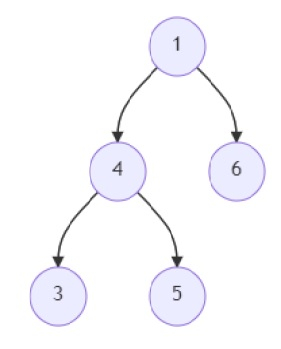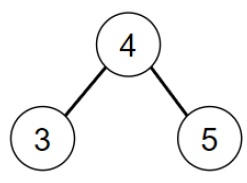# Program to find out if a BST is present in a given binary tree in Python

Suppose we are given a binary tree. We have to find out the largest subtree from the tree that is a binary search tree (BST). We return the root node of the BST.So, if the input is like

then the output will be −To solve this, we will follow these steps −

• c := 0
• m := null
• Define a function recurse() . This will take node
• if node is not null, then
• left_val := recurse(left of node)
• right_val := recurse(right of node)
• count := negative infinity
• if (node.left is same as null or node.left.val <= node.val) and (right of node is same as null or node.val <= node.right.val), then
• count := left_val + right_val + 1
• if count > c, then
• c := count
• m := node
• return count
• return 0
• recurse(root)
• return m

## Example

Let us see the following implementation to get better understanding −

class TreeNode:
def __init__(self, val, left = None, right = None):
self.val = val
self.left = left
self.right = right

def insert(temp,data):
que = []
que.append(temp)
while (len(que)):
temp = que
que.pop(0)
if (not temp.left):
if data is not None:
temp.left = TreeNode(data)
else:
temp.left = TreeNode(0)
break
else:
que.append(temp.left)

if (not temp.right):
if data is not None:
temp.right = TreeNode(data)
else:
temp.right = TreeNode(0)
break
else:
que.append(temp.right)
def make_tree(elements):
Tree= TreeNode(elements)
for element in elements[1:]:
insert(Tree, element)
return Tree

def print_tree(root):
if root is not None:
print_tree(root.left)
print(root.val, end = ', ')
print_tree(root.right)

def solve(root):
c, m = 0, None

def recurse(node):
if node:
nonlocal c, m
left_val = recurse(node.left)
right_val = recurse(node.right)
count = -float("inf")
if (node.left == None or node.left.val <= node.val) and (node.right == None or node.val <= node.right.val):
count = left_val + right_val + 1
if count > c:
c = count
m = node
return count
return 0

recurse(root)
return m

tree = make_tree([1, 4, 6, 3, 5])
print_tree(solve(tree))

## Input

tree = make_tree([1, 4, 6, 3, 5])
print_tree(solve(tree))

## Output

3, 4, 5,